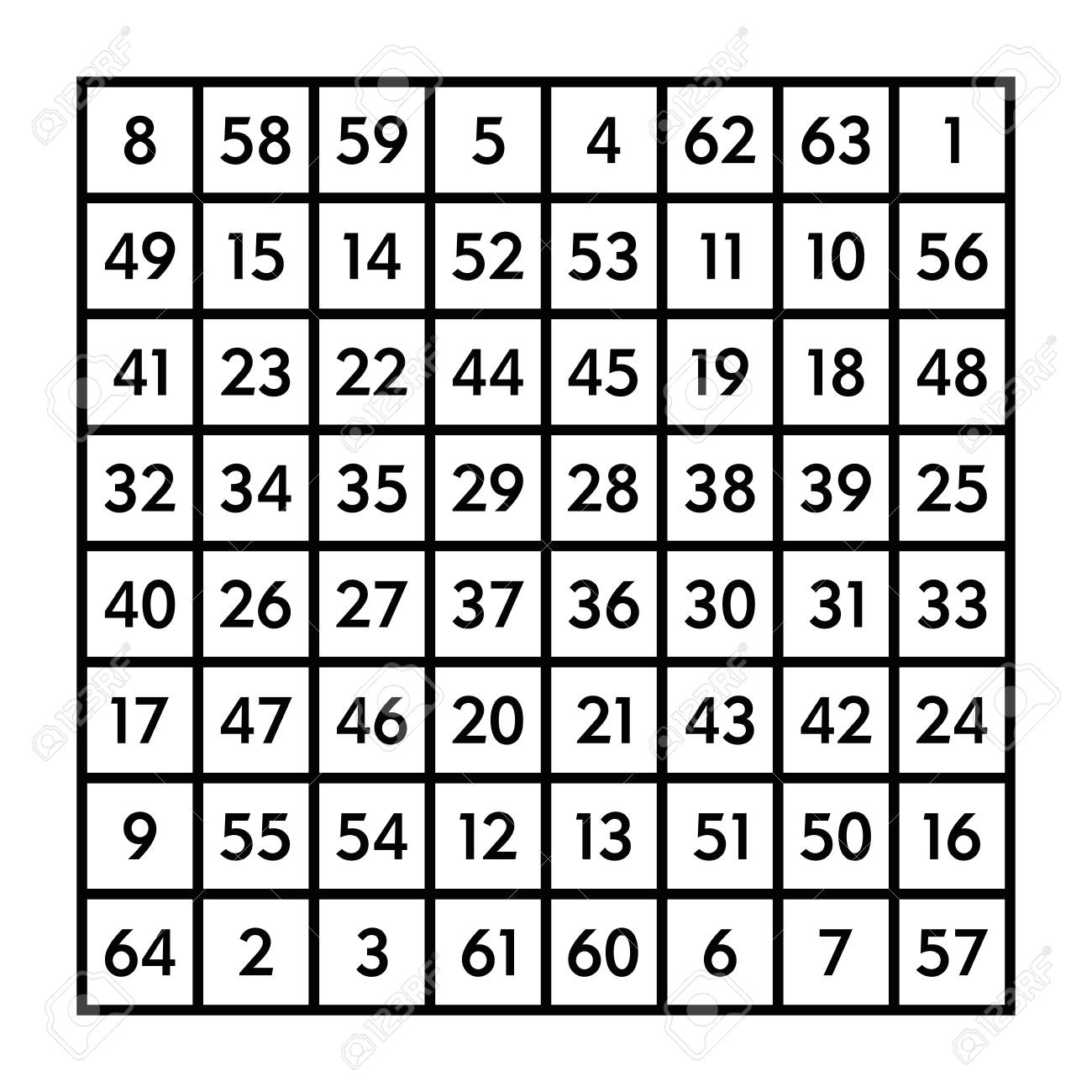# Two Famous Alternate Numbers, Here’s Why…

Two Famous Alternate Numbers, Here’s Why…What are these prominent famous ancient numbers?

• 2080 and 1080.

Most likely, at this point you are asking: How can they be alternate? They are a thousand numbers apart. After all, 2080 – 1080 = 1000!

John Michell, goes into the subject of these numbers in great detail. Actually, 1080 started me on a 32 year quest. John Frederick Carden Michell was an English author and esotericist,  He was a prominent in the Earth mysteries movement. Over his life he published over forty books.

### Two Famous Alternate Numbers Refer to the Same Thing

What is it that these two numbers refer to? Another, simpler number, being #8. Number “ten eighty” evokes the octagon. In our featured pictures the octagon has eight angles an 135°/each angle. Doing the math, 8 x 135° = 1080°.

### What about the ancient use of 2080?The Second famous alternate number is 2080. It totals the numbers from one to sixty-four.

How do we get 2080 out of this number square? Simply total all the numbers that it contains. The above picture is of the 8 x 8 magic square (also called a number square). Numbers are arranged so that any opposite two total 65.   Even though 1080 and 2080 are a thousand numbers apart, both of their essences invoke number eight according to the ancients.  One number, being 1080, conjures up geometry in the shape of an octagon. The other, being 2080, invokes a number square. Incidentally, this number square (or magic square) was supposed to invoke the influence of Mercury.

Here’s a mathematical shortcut to find 2080:  Any two opposite numbers total 65. It has 32 pairs of opposite numbers; therefore 32 x 65 =2080. Just how significant is this 8 x 8 grid? The greatest surviving man made wonder of the world is the Great Pyramid of Egypt. It is set on a grid that is 8 great cubits by 8 great cubits.

Here is an internal link on reviving antiquity.com: Pythagorean Triangle Numbers 3,4,5,